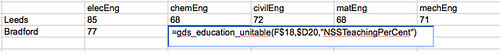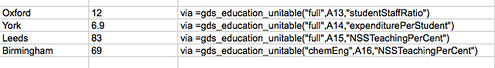# Grabbing “Facts” from the Guardian Datastore with a Google Spreadsheets Formula

In Using Data From Linked Data Datastores the Easy Way (i.e. in a spreadsheet, via a formula) I picked up on an idea outlined in Mulling Over =datagovukLookup() in Google Spreadsheets to show how to use Google Apps script to create a formula that could pull in live data from a data.gov.uk datastore.

So just because, here’s how to do something similar with data from a Google spreadsheet in the Guardian datastore. Note that what I’m essentially proposing here is using the datastore as a database…

To ground the example, consider the HE satisfaction tables:Lots of data about lots of courses on lots of sheets in a single spreadsheet. But how do you compare the satisfaction ratings across subjects for a couple of institutions? How about like this:(We can just click and drag the formula across a range of cells as we would any other formula.)

That is, how about defining a simple spreadsheet function that lets us look up a particular data value for a particular subject and a particular institution? How about being able to write a formula like:
=gds_education_unitable(“elecEng”,”Leeds”,”NSSTeachingPerCent”)
and get the national student satisfaction survey teaching satisfaction result back from students studying Electrical/Electronic Engineering at Leeds University?

Google Apps script provides a mechanism for defining formulae that can do this, and more:The script takes the arguments and generates a query to the spreadsheet using the spreadsheet’s visualisation API, as used in my Guardian Datastore Explorer. The results are pulled back as CSV, run through a CSV2Javacript object function and then returned to the calling spreadsheet. Here’s an example of the Apps script:

```function gds_education_unitable(sheet,uni,typ){
var key="phNtm3LmDZEM6HUHUnVkPaA";
var gid='0';//'Overall Institutional Table';
var category="C"; //(Average) Guardian teaching score
switch (sheet){
case "full":
gid='0';//'Overall Institutional Table';
break;
case "chemEng":
gid='16';//'15 Chem Eng';
break;
case "matEng":
gid='17';//'16 Mat Eng';
break;
case "civilEng":
gid='18';//'17 Civil Eng';
break;
case "elecEng":
gid='19';//'18 Elec Eng';
break;
case "mechEng":
gid='20';//'19 Mech Eng';
break;
default:
}

switch (typ){
case "guardianScore":
category='C';//(Average) Guardian teaching score
break;
case "NSSTeachingPerCent":
category='D';//
break;
case "expenditurePerStudent":
category='E';//
break;
case "studentStaffRatio":
category='F';//
break;
default:
}

if (sheet!='full') category=String.fromCharCode(category.charCodeAt(0)+2);

var x=UrlFetchApp.fetch(url);
var ret=x.getContentText();
ret = CSVToArray( ret, "," );
return ret;
}
```

(The column numbering between the first sheet in the spreadsheet and the subject spreadsheets is inconsistent, which is why we need a little bit of corrective fluff (if (sheet!=’full’)) in the code…)

Of course, we can also include script that will generate calls to other spreadsheets, or as I have shown elsewhere to other data sources such as the data.gov.uk Linked Data datastore.

Something that occurred to me though is if and how Google might pull on such “data formula” definitions to feed apps such as Google Squared (related: =GoogleLookup: Creating a Google Fact Engine Directory and Is Google Squared Just a Neatly Packaged and Generalised =googlelookup Array?).

## Author: Tony Hirst

I'm a Senior Lecturer at The Open University, with an interest in #opendata policy and practice, as well as general web tinkering...